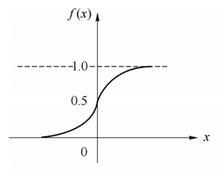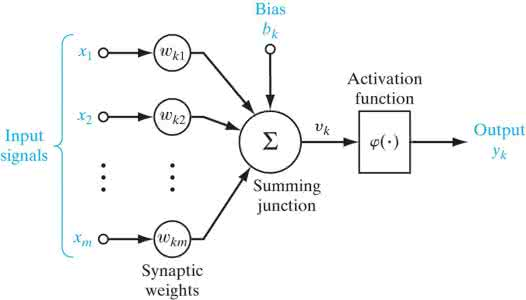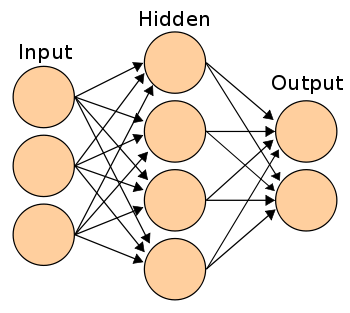神经网络基础知识XOR神经网络实现

<!DOCTYPE html>
<html>
<title>XOR神经网络实现</title>
<body>
<script src="https://cdnjs.cloudflare.com/ajax/libs/synaptic/1.1.0/synaptic.js"></script>
<script>
const { Network, Layer } = window.synaptic;
// 创建神经网络层
var inputLayer = new Layer(2); // 输入层
var hiddenLayer = new Layer(3); // 隐含层
var outputLayer = new Layer(1); // 输出层

// 搭建神经网络
inputLayer.project(hiddenLayer);
hiddenLayer.project(outputLayer);

var myNetwork = new Network({
input: inputLayer,
hidden: [hiddenLayer],
output: outputLayer
});

// 神经网络训练
var train = [[0,0], [0,1], [1,0], [1,1]]; // 训练数据
var labels = [0, 1, 1, 0]; // 标签
var learningRate = 0.1; // 学习率

// 将训练数据10000次
for (var i = 0; i < 10000; i++) {
for(var j = 0; j < train.length; j++) {
myNetwork.activate(train[j]);
myNetwork.propagate(learningRate, [labels[j]]);
}
}

// 测试输出
for(var j = 0; j < train.length; j++) {
console.log(train[j] + "-> predict: " + myNetwork.activate(train[j]) + "; label："+labels[j]);
}
</script>
</body>
</html>

0,0-> predict: 0.0005624499128064958; label：0
test.html:39 0,1-> predict: 0.9970162975810412; label：1
test.html:39 1,0-> predict: 0.9971341382083764; label：1
test.html:39 1,1-> predict: 0.004872445055285009; label：0

代码解析

创建神经网络层

const { Network, Layer } = window.synaptic;
// 创建神经网络层
var inputLayer = new Layer(2); // 输入层
var hiddenLayer = new Layer(3); // 隐含层
var outputLayer = new Layer(1); // 输出层

神经网络的搭建

// 搭建神经网络
inputLayer.project(hiddenLayer);
hiddenLayer.project(outputLayer);

var myNetwork = new Network({
input: inputLayer,
hidden: [hiddenLayer],
output: outputLayer
});

神经网络的训练

// 神经网络训练
var train = [[0,0], [0,1], [1,0], [1,1]]; // 训练数据
var labels = [0, 1, 1, 0]; // 标签
var learningRate = 0.1; // 学习率

// 将训练数据10000次
for (var i = 0; i < 10000; i++) {
for(var j = 0; j < train.length; j++) {
myNetwork.activate(train[j]);
myNetwork.propagate(learningRate, [labels[j]]);
}
}